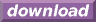#Calcute: freeware calculator

## Square Root (sqrt)

The square root of a value is this value raised to the power of 1/2. Calcute implements this operation with the sqrt function. The same result is obtained either through the sqrt function or using the power operator ^ with the value of one half, so sqrt(x) = x^1\2.

```sqrt(9)
3
9^1\2
3
```

Note on notation: Calcute always understands simple ratios that are expressed using a back slash character, as shown above. A user-set option also allows restricted use of the forward slash (division operator) for the same purpose, but it is recommended to use the back slash in order to avoid ambiguity between these different uses.